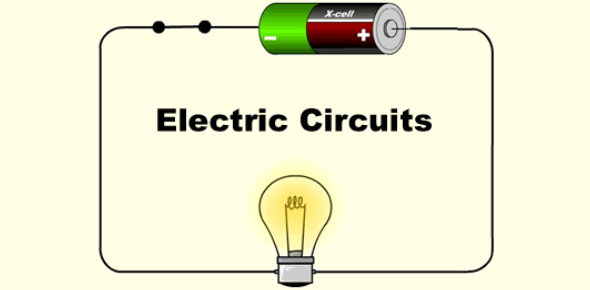# Electrical Circuits Exam! 5th Grade Science Quiz

20 Questions | Total Attempts: 5842SettingsAre you someone who wants to learn more about the basics electrical circuits? Would you be willing to try this quiz for fifth-graders? In this quiz, you will be responsible for knowing why Christmas lights connected in a series are not a good idea, an energy storage device made of electrochemical cells, and the primary energy transfer in a lamp. If you are searching for more information about electrical circuits, look no further than this quiz.

• 1.
Christmas tree lights are often connected in series. This is not a good idea because
• A.

They use more energy

• B.

They aren't colorful

• C.

If one light breaks they all go out

• D.

There isn't a complete circuit

• 2.
What is an energy storage device composed of electrochemical cells?
• A.

A fuse

• B.

A flashlight

• C.

A battery

• D.

A circuit

• 3.
What is the name of this piece of equipment?
• A.

A resistor

• B.

An open switch

• C.

Two light bulbs

• D.

Closed Switch

• 4.
What is the main energy transfer in a lamp?
• A.

Light to electrical

• B.

Heat to light

• C.

Electrical to chemical

• D.

Electrical to light

• 5.
This is the circuit symbol for which piece of electrical equipment?
• A.

A battery

• B.

A switch

• C.

A wire

• D.

A cell

• 6.
Electricity flows through a wire. We call this a current. Current is measured in what units?
• A.

Joules

• B.

Amps

• C.

Volts

• D.

Watts

• 7.
All lamps have some resistance.  Putting a lamp with large resistance into a circuit will
• A.

Make the voltage bigger

• B.

Make the voltage smaller

• C.

Make the current smaller

• D.

Make the current bigger

• 8.
You have identical lamps and cells, which combination gives the brightest circuit?
• A.

Two cells connected to one lamp

• B.

Two cells connected to two lamps in a series

• C.

Two lamps in parallel series connected to one cell

• D.

One cell connected to one lamp

• 9.
Two lamps are connected side-by-side so that the current is shared between them. We call this connected.
• A.

In a series

• B.

In a parallel

• C.

In a circle

• D.

In a loop

• 10.
Lamps in your house are often connected in parallel.  This is a good idea because
• A.

They are cheaper

• B.

They use less current

• C.

You can switch them off separately

• D.

The lights are dimmer

• 11.
In the picture, what does the short line represent?
• A.

A battery

• B.

Electron portion of battery

• C.

Electron portion of cell

• D.

Proton portion of cell

• 12.
A series circuit has one path from the source and back to the source again.
• A.

True

• B.

False

• 13.
If you unscrew one of the light bulbs in a parallel circuit, the rest will stop lighting.
• A.

True

• B.

False

• 14.
Glass is a conductor of electricity.
• A.

True

• B.

False

• 15.
Electricity is a force?
• A.

True

• B.

False

• 16.
Electric current gets used up in a circuit?
• A.

True

• B.

False

• 17.
Electrical current is the flow of electrical charges (electrons) around a circuit?
• A.

True

• B.

False

• 18.
The more bulbs you add to a circuit, the more difficult it will be for the current to flow?
• A.

True

• B.

False

• 19.
Which group of items are conductors?
• A.

A

• B.

B

• C.

C

• D.

D

Related TopicsBack to top